### ORAnGE (revisited)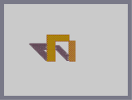Hover over the thumbnail for a full-size version.

Author killn2 author:killn2 n-art nonplayable orange rated 2008-02-28 4 by 5 people. \$ORAnGE (revisited)#killn2#none#00000000000000000000000000000000000000000000000000000000000000000000000000000000000000000000000000000000000000000000000000000000000000000000000000000000000000000000000000000000000000000000000000000000000000000000000000000000000000000000000000000000000000000000000000000000000000000000000000000000000000000000000000000000000000000000000000000000000000000000000000000000000000000000000000000000000000000000000000000000000000000000000000000000000000000000000000000000000000000000000000000000000000000000000000000000000000000000000000000000000000000000000000000000000000000000000000000000000000000000000000000000000000000000000000000000000000000000000000000000000000000000000000000000000000000000000000000000000000000|0^282,354!0^282,342!0^282,330!0^282,318!0^282,306!0^282,294!0^282,282!0^282,270!0^282,258!0^282,246!0^282,234!0^282,222!0^282,210!0^294,210!0^306,210!0^318,210!0^330,210!0^342,210!0^354,210!0^366,210!0^378,210!0^390,210!0^402,210!0^414,210!0^414,222!0^414,234!0^414,246!0^402,246!0^390,246!0^378,246!0^366,246!0^354,246!0^342,246!0^330,246!0^330,258!0^330,270!0^330,282!0^330,294!0^330,306!0^330,318!0^330,330!0^330,342!0^330,354!0^330,366!0^318,366!0^306,366!0^294,366!0^414,258!0^414,270!0^414,282!0^414,294!0^414,306!0^414,318!0^414,330!0^414,342!0^414,354!0^426,366!0^438,366!0^450,366!0^450,354!0^450,342!0^450,330!0^450,318!0^450,306!0^450,294!0^450,282!0^450,270!0^450,258!0^450,246!0^438,246!0^426,246!0^420,252!0^432,252!0^444,252!0^444,264!0^438,258!0^426,258!0^420,264!0^432,264!0^426,270!0^438,270!0^444,276!0^432,276!0^420,276!0^426,282!0^438,282!0^444,288!0^432,288!0^420,288!0^420,300!0^426,294!0^432,300!0^438,294!0^444,300!0^426,306!0^438,306!0^444,312!0^432,312!0^420,312!0^426,318!0^438,318!0^444,324!0^432,324!0^420,324!0^426,330!0^438,330!0^444,336!0^432,336!0^420,336!0^426,342!0^438,342!0^444,348!0^432,348!0^420,348!0^426,354!0^438,354!0^444,360!0^432,360!0^420,360!12^414,360!12^414,348!12^414,336!12^414,324!12^414,312!12^414,300!12^414,288!12^414,276!12^414,264!12^414,252!12^420,246!12^432,246!12^444,246!12^450,252!12^450,264!12^450,276!12^450,288!12^450,300!12^450,312!12^450,324!12^450,336!12^450,348!12^450,360!12^444,366!12^432,366!12^420,366!12^420,354!12^432,354!12^426,360!12^438,360!12^444,354!12^438,348!12^426,348!12^420,342!12^432,342!12^444,342!12^438,336!12^426,336!12^420,330!12^432,330!12^444,330!12^438,324!12^426,324!12^420,318!12^432,318!12^444,318!12^438,312!12^426,312!12^420,306!12^432,306!12^444,306!12^438,300!12^426,300!12^420,294!12^432,294!12^444,294!12^438,288!12^426,288!12^420,282!12^432,282!12^444,282!12^438,276!12^426,276!12^420,270!12^432,270!12^444,270!12^438,264!12^426,264!12^420,258!12^432,258!12^444,258!12^438,252!12^426,252!12^414,240!12^414,228!12^414,216!12^408,210!12^396,210!12^384,210!12^372,210!12^360,210!12^348,210!12^336,210!12^324,210!12^312,210!12^300,210!12^288,210!12^282,216!12^282,228!12^282,240!12^282,252!12^282,264!12^282,276!12^282,288!12^282,300!12^282,312!12^282,324!12^282,336!12^282,348!12^282,360!12^288,366!12^300,366!12^312,366!12^324,366!12^330,360!12^330,348!12^330,336!12^330,324!12^330,312!12^330,300!12^330,288!12^330,276!12^330,264!12^330,252!12^336,246!12^348,246!12^360,246!12^360,246!12^372,246!12^384,246!12^396,246!12^408,246!12^324,354!12^312,354!12^300,354!12^288,354!12^294,360!12^306,360!12^318,360!12^318,348!12^306,348!12^294,348!12^288,342!12^300,342!12^312,342!12^324,342!12^318,336!12^306,336!12^294,336!12^288,330!12^300,330!12^312,330!12^324,330!12^318,324!12^306,324!12^294,324!12^288,318!12^300,318!12^312,318!12^324,318!12^318,312!12^306,312!12^294,312!12^288,306!12^300,306!12^312,306!12^324,306!12^318,300!12^306,300!12^294,300!12^288,294!12^300,294!12^312,294!12^324,294!12^318,288!12^306,288!12^294,288!12^288,282!12^300,282!12^312,282!12^324,282!12^318,276!12^306,276!12^294,276!12^288,270!12^300,270!12^312,270!12^324,270!12^318,264!12^306,264!12^294,264!12^288,258!12^300,258!12^312,258!12^324,258!12^318,252!12^324,246!12^312,246!12^306,240!12^300,234!12^294,228!12^288,222!12^294,216!12^300,222!12^306,228!12^312,234!12^318,240!12^324,246!12^306,252!12^300,246!12^294,240!12^288,234!12^288,246!12^294,252!12^306,216!12^312,222!12^324,234!12^330,240!12^318,216!12^324,222!12^330,228!12^336,234!12^348,234!12^342,228!12^330,216!12^336,222!12^342,240!12^354,240!12^366,240!12^378,240!12^390,240!12^402,240!12^408,234!12^396,234!12^384,234!12^372,234!12^360,234!12^354,228!12^348,222!12^342,216!12^354,216!12^366,216!12^378,216!12^390,216!12^402,216!12^408,222!12^402,228!12^396,222!12^390,228!12^384,222!12^378,228!12^372,222!12^366,228!12^360,222!0^324,360!0^312,360!0^300,360!0^288,360!0^288,348!0^294,354!0^300,348!0^306,354!0^312,348!0^318,354!0^324,348!0^318,342!0^306,342!0^294,342!0^288,336!0^300,336!0^312,336!0^324,336!0^318,330!0^306,330!0^294,330!0^288,324!0^300,324!0^312,324!0^324,324!0^318,318!0^306,318!0^294,318!0^288,312!0^300,312!0^312,312!0^324,312!0^318,306!0^306,306!0^294,306!0^288,300!0^294,294!0^300,300!0^306,294!0^312,300!0^318,294!0^324,300!0^324,288!0^312,288!0^300,288!0^288,288!0^294,282!0^306,282!0^318,282!0^324,276!0^312,276!0^300,276!0^288,276!0^294,270!0^306,270!0^318,270!0^324,264!0^312,264!0^300,264!0^288,264!0^294,258!0^306,258!0^318,258!0^324,252!0^312,252!0^300,252!0^288,252!0^318,246!0^306,246!0^294,246!0^288,240!0^294,234!0^288,228!0^288,216!0^300,216!0^294,222!0^306,222!0^306,234!0^300,228!0^300,240!12^318,228!0^312,240!0^324,240!0^336,240!0^348,240!0^360,240!0^372,240!0^384,240!0^396,240!0^408,240!0^408,228!0^408,216!0^396,216!0^384,216!0^372,216!0^360,216!0^348,216!0^336,216!0^324,216!0^312,216!0^318,222!0^330,222!0^342,222!0^354,222!0^366,222!0^378,222!0^390,222!0^402,222!0^396,228!0^384,228!0^372,228!0^360,228!0^348,228!0^336,228!0^324,228!0^312,228!0^318,234!0^330,234!0^342,234!0^354,234!0^366,234!0^378,234!0^390,234!0^402,234!3^246,330!3^240,324!3^234,318!3^228,312!3^222,306!3^210,294!3^216,300!3^204,288!3^198,282!3^192,276!3^186,270!3^180,264!3^186,264!3^192,264!3^198,264!3^204,264!3^210,264!3^216,264!3^222,264!3^222,264!3^198,282!3^210,294!3^234,264!3^228,264!3^240,264!3^240,264!3^246,264!3^252,264!3^258,264!3^258,264!3^264,264!3^264,264!3^264,264!3^270,264!3^276,264!3^270,264!3^408,330!3^402,324!3^396,318!3^390,312!3^384,306!3^378,300!3^372,294!3^366,288!3^276,318!3^270,312!3^264,306!3^258,300!3^252,294!3^258,294!3^270,294!3^270,294!3^276,294!3^360,282!3^360,282!3^354,276!3^402,360!3^396,354!3^390,348!3^384,342!3^378,336!3^372,330!3^366,324!3^360,318!3^354,312!3^408,360!3^348,306!3^342,300!3^336,294!3^348,276!3^336,276!3^342,276!0^282,366!3^252,336!3^258,342!3^264,348!3^270,354!3^276,360!3^270,348!3^264,336!3^264,336!3^258,330!3^252,330!3^246,324!3^240,318!3^240,312!3^234,312!3^228,306!3^228,300!3^228,300!3^216,294!3^216,288!3^210,282!3^210,276!3^198,276!3^204,276!3^204,276!3^210,276!3^210,276!3^216,276!3^222,276!3^234,276!3^234,276!3^234,276!3^246,276!3^246,276!3^258,276!3^264,276!3^264,276!3^270,276!3^276,276!3^264,276!3^264,276!3^258,276!3^252,276!3^252,276!3^252,276!3^246,270!3^246,276!3^246,276!3^252,276!3^240,276!3^234,270!3^228,270!3^222,276!3^228,276!3^234,276!3^234,276!3^252,282!3^258,282!3^252,312!3^246,306!3^240,300!3^234,294!3^228,294!3^228,288!3^222,282!3^216,282!3^222,282!3^240,282!3^246,288!3^246,288!3^246,288!3^240,288!3^228,282!3^222,282!3^216,282!3^210,282!3^204,282!3^192,270!3^192,270!3^192,264!3^192,270!3^192,270!3^198,270!3^204,270!3^210,270!3^210,270!3^216,270!3^222,270!3^228,270!3^240,270!3^240,306!3^240,306!3^246,312!3^252,312!3^252,312!3^240,306!3^234,300!3^234,294!3^240,294!3^240,300!3^240,306!3^240,288!3^240,282!3^228,282!3^222,288!3^222,300!3^270,348!3^270,342!3^270,336!3^270,336!3^270,330!3^270,330!3^270,324!3^276,330!3^276,330!3^276,336!3^276,342!3^276,348!3^276,354!3^276,354!3^276,324!3^270,324!3^264,312!3^258,306!3^258,306!3^252,306!3^246,300!3^252,306!3^264,324!3^264,324!3^264,324!3^258,318!3^252,318!3^252,318!3^252,318!3^252,324!3^258,324!3^258,318!3^258,318!3^258,330!3^258,336!3^264,342!3^264,336!3^264,318!3^264,324!3^264,324!3^264,330!3^270,318!3^270,312!3^258,294!3^252,288!3^240,276!3^234,270!3^234,276!3^240,288!3^240,288!3^210,288!3^216,288!3^222,288!3^228,288!3^228,288!3^228,288!3^234,288!3^234,288!3^234,288!3^234,288!3^240,288!3^240,288!3^240,288!3^246,294!3^246,294!3^246,294!3^252,294!3^258,294!3^264,294!3^264,294!3^264,294!3^270,294!3^270,294!3^270,294!3^222,300!3^228,300!3^228,300!3^240,300!3^240,300!3^240,300!3^240,300!3^246,300!3^246,300!3^252,300!3^252,300!3^258,300!3^258,300!3^222,294!3^222,294!3^234,294!3^246,282!3^234,282!3^246,318!3^234,306!3^258,312!3^252,270!3^264,282!3^270,282!3^252,270!3^264,270!3^264,270!3^264,270!3^270,270!3^258,270!3^276,270!3^276,270!3^276,288!3^270,288!3^264,288!3^264,288!3^264,288!3^264,282!3^258,288!3^276,288!3^336,282!3^336,282!3^342,282!3^348,282!3^348,282!3^354,288!3^354,288!3^348,288!3^342,288!3^342,288!3^366,312!3^366,312!3^372,324!3^372,330!3^390,336!3^402,348!3^402,348!3^408,348!3^402,336!3^396,330!3^396,330!3^390,324!3^372,318!3^372,312!3^372,312!3^372,306!3^366,300!3^354,294!3^354,294!3^360,300!3^360,306!3^378,312!3^384,324!3^384,324!3^384,330!3^384,330!3^390,336!3^396,342!3^402,342!3^402,342!3^396,336!3^384,324!3^384,324!3^378,318!3^378,312!3^360,294!3^348,288!3^354,294!3^354,300!3^354,300!3^354,300!3^360,306!3^366,312!3^348,294!3^360,312!3^354,312!3^354,306!3^348,306!3^348,306!3^348,294!3^348,300!3^342,300!3^360,318!3^366,324!3^360,318!3^366,318!3^354,312!3^348,306!3^336,294!3^342,294!3^342,288!3^342,288!3^336,282!3^336,282!3^378,336!3^390,342!3^396,342!3^396,342!3^396,342!3^378,330!3^378,330!3^378,330!3^390,318!3^372,306!3^360,300!3^372,306!3^378,312!3^378,312!3^378,300!3^366,294!3^366,294!3^366,288!3^402,342!3^390,330!3^378,318!3^378,318!3^378,318!3^378,306!3^378,306!3^378,300!3^372,300!3^372,294!3^354,282!3^360,282!3^360,282!3^396,342!3^390,336!3^384,330!3^384,324!3^378,318!3^378,318!3^366,306!3^384,318!3^384,318!3^384,324!3^384,324!3^378,330!3^360,288!3^408,336!3^396,330!3^390,324!3^384,318!3^384,312!3^378,324!3^402,330!3^402,348!3^402,348!3^402,354!3^396,348!3^390,342!3^390,342!3^384,336# added shading and took away the background.

## Other maps by this author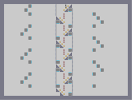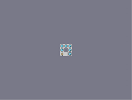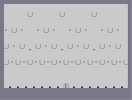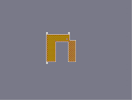A thwumpy tower E - Z Challenge 4 (tweeked) Nball oraNge